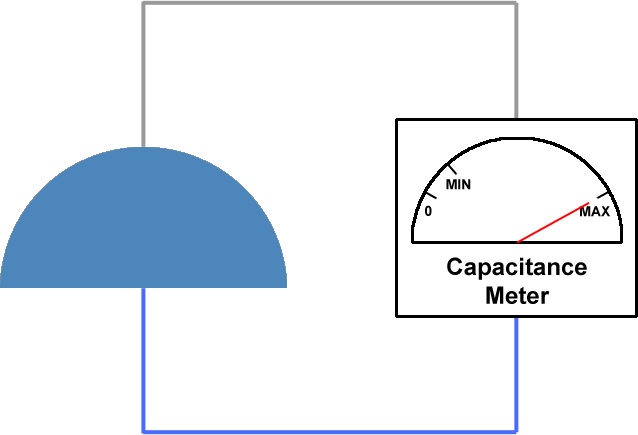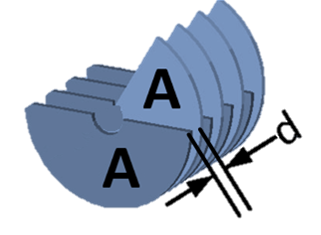## What are air capacitors?Air capacitors are capacitors which use air as their dielectric. The simplest air capacitors are made of two conductive plates separated by an air gap. Air capacitors can be made in a variable or fixed capacitance form. Fixed capacitance air capacitors are rarely used since there are many other types with superior characteristics. Variable air capacitors are used more often because of their simple construction. They are usually made of two sets of semicircular metal plates separated by air gaps. One set is fixed and the other is attached to a shaft which allows the user to rotate the assembly, therefore changing the capacitance as needed. The larger the overlap between the two sets of plates, the higher the capacitance. The maximum capacitance state is achieved when the overlap between the two sets of plates is highest, while the lowest capacitance state is achieved when there is no overlap.Variable air capacitors are used in circumstances where the capacitance needs to be varied. They are sometimes used in resonant circuits, such as radio tuners, frequency mixers or antenna impedance matching applications. Another use for variable capacitors is while prototyping an electronic circuit design. Sometimes, it is simply easier to find the appropriate capacitor value by trial and error instead of calculating it exactly.

## Air capacitor definition

Air capacitors are capacitors which use air as the dielectric medium located between conductive plates.

## Characteristics

The dielectric constant value of a material is a measure of the amount of electrical energy stored in a material for a given voltage. Since capacitors are devices used to store electrical energy, higher dielectric constants are favorable. However, the dielectric value of air is approximately that of vacuum, which is the lowest dielectric constant possible for a material and equals ε= 8.854 * 10-12 F/m. This means that in order to achieve large capacitance values, a physically large capacitor is needed. Another important factor is the dielectric strength. The dielectric strength limits the maximum voltage that can be applied to a material before it loses its insulator and dielectric properties and starts acting like a conductor. The dielectric strength of air is inferior to many other materials, which makes air capacitors unsuitable for high voltages.

Air capacitors have a small capacitance which usually lies between 100pF and 1nF. The maximum working voltage depends on the physical dimensions of the capacitor. A high operating voltage requires that the distance between plates is sufficient to avoid electrical breakdown of air. If breakdown occured, it would cause sparking between the plates, the capacitor would not work properly and could even get damaged by the electrical arc. Therefore, capacitors that work at higher voltages are generally physically larger to allow sufficient space between plates. Typical operating voltages are between a few tens to a few thousands of volts. For higher operating voltages, a similarly constructed capacitor is sometimes used, however air is evacuated from the device and vacuum is used as the dielectric. The dielectric constant of vacuum is nearly the same as that of air, but the breakdown voltage is higher and not dependent on air humidity and particulate pollution.

Air capacitors are non-polar. This means that air capacitors may be safely used without regard to DC polarity, which is useful in some applications. They are safe for use in AC applications, as long as the maximum voltage rating is not exceeded.

## Construction and properties of air capacitorsVariable air gap capacitors are usually made of two groups of semicircular metal plates. One group is fixed, while the other can be rotated using a shaft connected to it. The two groups are sandwiched with an air gap between each two plates. The user changes the capacitance by rotating the shaft and increasing or reducing the amount of overlap between the two groups of plates. Reduction gear mechanisms may be used to increase the precision and allow for finer tuning and better capacitance control. By carefully engineering the shape of the plates, it is possible to achieve, for example, a linear or logarithmic function of angle of rotation vs. capacitance. In order to improve the characteristics of air capacitors, sometimes the air is evacuated from a sealed chamber and this creates a vacuum capacitor. Other materials, such as plastic foils may also be used as the dielectric material.

In spite of the relatively low breakdown voltage and low dielectric constant of air and vacuum, they still have some advantages over other materials. The most important advantage is the low leakage current, meaning that the operating losses inside an air capacitor are minimal, especially if the humidity is low.

### Multiple sections variable capacitor

There are several special types of variable capacitors considering their construction. One type is the multiple section variable capacitor, which has two or more capacitors of same or different capacitances connected to the same shaft. Rotating the control shaft will affect the capacitances of all the capacitors on it and through careful design it is possible to use one variable capacitor to tune several circuits at the same time, such as a preselector, an input filter and the oscillator in a receiver unit.

### Butterfly variable capacitor

Butterfly variable capacitors have two stators and a rotor arranged in such a way that turning the rotor will vary the capacitance between the rotor and either stator equally.

### Differential variable capacitor

Differential variable capacitors also use two stators, however rotating the control shaft increases capacitance on one stator while reducing the capacitance on the other. The capacitance between two stators is thus held constant.

## Applications for variable capacitors

Applications for variable capacitors are mostly constrained to AC circuits. Most applications demand high frequency, high power and low loss properties. They are used in radio tuning applications, antenna tuning and so on. Air capacitors are interesting for military applications due to their inherent resistance to electromagnetic pulses which can be created intentionally to destroy electronic equipment, but are also a byproduct of a nuclear explosion. Modern day applications include RF matching networks for plasma control in conductive material deposition on silicon wafers, as well as MRI medical scanners, which require non-magnetic variable capacitors. Since air capacitors are made of aluminum, which is non-magnetic, they work great in strong magnetic fields.